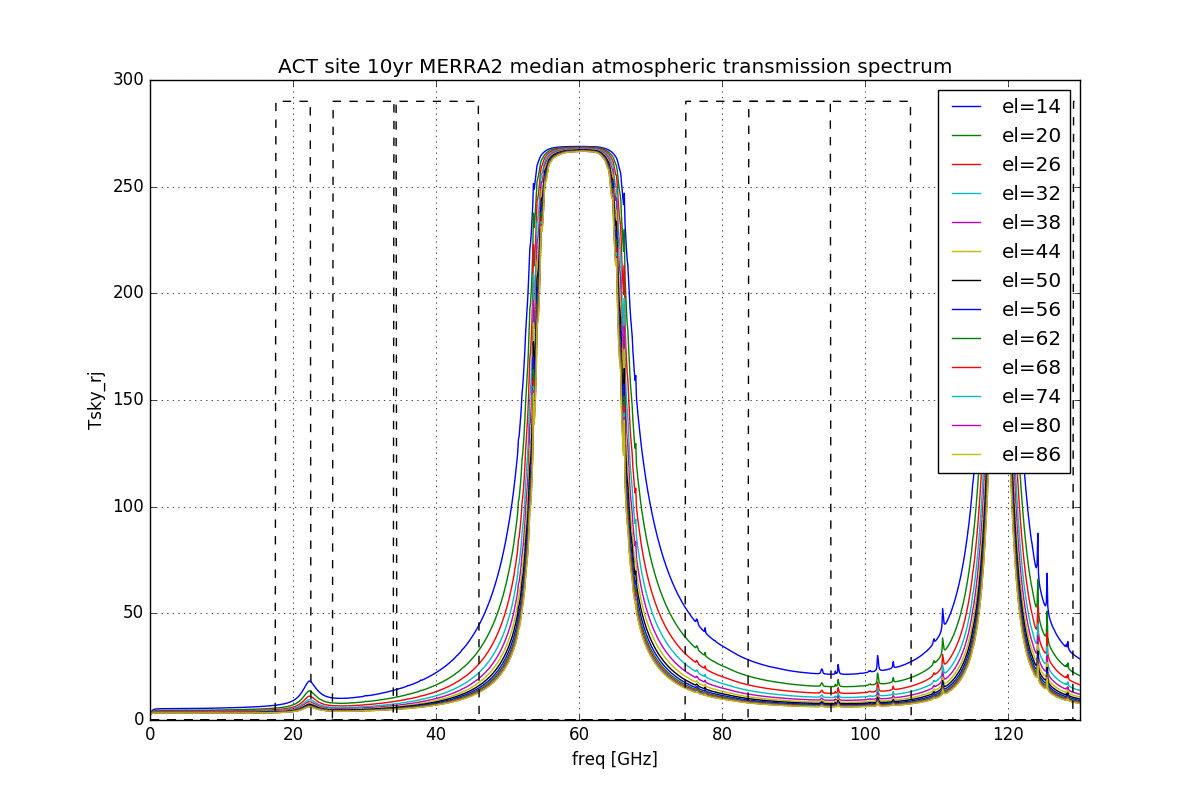# CMBS4 NET forecasts follow-up II

Denis Barkats
Updated by Ben Racine for bath temperature T0 = 100 mK results (2019-02-25).
Updated by Ben Racine to provide a cosecant+offset fit (2019-03-21).

### Summary

Following up on a suggestion from Clem to plot the predicted NET for a standard B3-like instrument, as a function of observing elevation to see the price you pay in NET as you observe lower.

I did this by using the newly developed python NET calculator (see details here). We use the now-standard 10yr-median MERRA atmospheric profile for both South Pole site and ACT site (lon=-67.788°, lat=-22.959°, alt=5190m), and allow am to calculate the atmospheric transmission at elevations from el=14 to el=90deg (Note: This option not yet deployed on the web version of the NET calculator). Then I simply use these atmospheric transmission spectra at different elevation to calculate the NET for the standard 8+1 CMBS4 standard bandpasses (20/0.25, 30/0.3, 40/0.3, 85/0.24, 95/0.24, 145/0.22, 155/0.22, 220/0.22, 270/0.22). All other inputs remain fixed (ie. T0 = 250mK -- Now also including 100mK).
Note that in the previous time series posting, we used the position at the Chajnantor Plateau (ALMA) site (lon=-67.76175°,lat=-23.0285°,alt=5060m), and not the ACT site.

### Results

Jumping right away to the main result plot, I show the NET vs elevation for the 9 bandpasses. Note the y-axis range. South Pole NETs in dashed, CP in solid.

The second plot below shows the NET renormalized to value of the NET for South Pole and el=56deg. It shows that the largest difference between SP and CP is for the 40GHz band, followed by the 85 and 95GHz. I am guessing this is because those are the bands which are closest to the 60GHz oxygen line in that order and this reflects the more than 2000m difference between the CP site and the SP site.Figure 1: NET as a function of elevation for South Pole (dashed) and Chile (solid), for two different detector bath temperature.

To confirm that the 40GHz band is indeed the most affected by the relative altitude of the two sites, I plot the actual atmospheric spectra use to derive the NETS at the various elevations, at SP and CP sites. The band edges are overlaid. Below is an animated gif flipping between the 2 spectra. You can see that the CP site has higher Trj in the saturated part of the 60GHz emission line than the SP site (270K vs 230K). That's just the physical temperature of the atmosphere at those 2 sites. However, the lower pressure at the CP sites yields lower pressure broadening and thus lower temperature in the wings at CP than at SP. This goes to show again that pwv is *NOT* the only relevant parameter when comparing one site to the other.Figure 2: Rayleigh-Jeans temperature of the sky a both sites.

### Functional form of the elevation dependence.

Here we report the fit of a cosecant + offset model of the elevation dependence: f(el) = a / sin(el) + b, shown in figure 3.
The fit parameters as well as a small python plotting script is provided here:

### Plotting script for the fit

    import numpy as np
from matplotlib import pyplot as plt
# define frequency band names
bandNames = ['20','30','40','85','95','145','155','220','270']

# function that was used for the fit
def csc_off(x, a, b):
return a / np.sin(x) + b

# elevation of the data points used for the fit
el = np.arange(14,92,2)

# (a,b) parameters for the 9 frequencies (T_0=100mK)
params_Chile=np.array([[ 33.72945249, 178.59719595],[ 28.66931669, 138.33886592],[ 66.45668561, 122.56542444],[ 77.42767099, 148.16640151],[ 57.69016952, 150.67611435],[ 69.37695133, 216.24466349],[ 85.42724656, 226.9066298 ],[227.4301014 , 484.95996316],[473.37864377, 738.31550923]])
params_Pole=np.array([[ 37.3914158 , 179.22330615],[ 47.82492978, 138.0087745 ],[120.99303676, 118.2242207 ],[135.83053702, 142.14623313],[ 94.79121048, 148.43263742],[ 83.90223764, 215.51967242],[ 88.58942678, 226.92306915],[218.06191292, 485.93308871],[454.06683583, 740.33536948]])

# here is for T_0=250mK
#params_Pole=np.array([[ 39.1900006 , 232.15336395],[ 50.14873321, 174.37731335],[124.48393518, 150.78451392],[139.72207723, 169.5567528 ],[ 98.22021733, 173.95680569],[ 87.09041062, 242.30301058],      [ 91.91255716, 254.15203494],[224.1987897 , 528.87316188],[465.5690534 , 801.04772039]])
#params_Chile=np.array([[ 35.18313617, 231.5258254 ],[ 30.3459686 , 174.0583013 ], [ 69.01306803, 153.64517758], [ 80.29111111, 174.2137814 ],[ 60.25763568, 175.11559628],[ 72.21118082, 242.65622106],[ 88.5873256 , 254.06694633],[233.74328147, 528.01034797],[485.15407612, 799.2362624 ]])

plt.figure(1, figsize=(12,8));plt.clf()
for i in range(0,9):
plt.plot(el,csc_off(el*np.pi/180.,params_Pole[i,0],params_Pole[i,1]),'-')
plt.legend(bandNames[0:9])
plt.xlabel('elevation [deg]')
plt.ylabel('NET [uKrts]')
plt.suptitle('Pole NET vs elevation (fit), T0=100mK, nominal instrument')

plt.figure(2, figsize=(12,8));plt.clf()
for i in range(0,9):
plt.plot(el,csc_off(el*np.pi/180.,params_Chile[i,0],params_Chile[i,1]),'-')
plt.legend(bandNames[0:9])
plt.xlabel('elevation [deg]')
plt.ylabel('NET [uKrts]')
plt.suptitle('Chile (ACT site) NET vs elevation (fit), T0=100mK, nominal instrument')Figure 3: Elevation dependence of the NET (colored dots) and its cosecant fit (black solid lines), for $$T_0= 100 mK$$